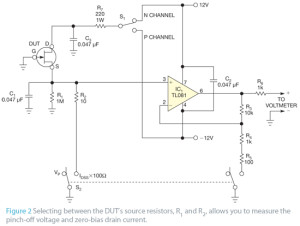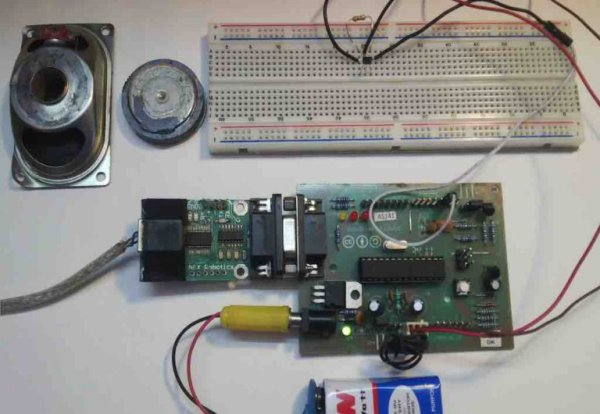# Simple circuit lets you characterize JFETs

When working with discrete JFETs, designers may need to accommodate a large variation in device parameters for a given transistor type. A square-law equation is usually used as an approximate model for the drain-current characteristic of the JFET: ID=β(VGS−VP)2, where ID is the drain current, VGS is the gate-to-source voltage, β is the transconductance parameter, and VP is the gate pinch-off voltage. With this approximation, the following equation yields the zero-bias drain current at a gate-to-source voltage of 0V: IDSS=βVP 2, where IDSS is the zero-bias drain current.

Although you can design around a certain amount of device variation for a mass-produced circuit, you sometimes need a tool to quickly characterize an assortment of discrete devices. This tool allows you to select a device that will optimize one circuit or perhaps to find a pair of devices with parameters that match reasonably well.Figure 2 shows a simple test circuit for this purpose. Although the figure shows the JFET as an N-channel device, the JFET DUT (device under test) may be of either polarity, as selected by switch S1. An external voltmeter connects to the terminals on the right. Switch S2 selects two distinct measurement modes—one for the pinch-off voltage and another for the zero-bias drain current. In the pinch-off-voltage mode, the external voltmeter directly reads the pinch-off voltage; in the zero-bias-drain-current mode, the measured voltage is the zero-bias drain current across an apparent resistance of 100Ω.

With S2 in the pinch-off-voltage mode, R1 allows a few microamps of drain current to flow in the JFET under test, and the source voltage is a close approximation of the negative of the pinch-off voltage. The op amp acts as a unity-gain buffer, with negative feedback through R3, so you can directly read the negative of the pinch-off voltage with the external voltmeter.

READ  How to Build a Vibration Detector Circuit

For More Details: Simple circuit lets you characterize JFETs